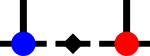SyTen◆ operator>>() [5/6]

template<typename Scalar >
 std::istream& syten::operator>> ( std::istream & in, DenseTensor< 2, Scalar > & v )
inline

Parse a rank-2 tensor from an input stream.

Only use this when text files are absolutely necessary, normally, use the serialisation functions.

Input format is the same as the output format generated by operator<<(std::ostream&, DenseTensor<2, Scalar>&), i.e.:

(-1.70921,0.793797) (0.562942,0.573431) (-0.841204,1.15254)

for a rank-2 tensor of 2 × 3 complex elements. Note that anything that can be parsed into the scalar type of the tensor is accepted there.

References read(), std::istream::setstate(), and std::stol().Here is the call graph for this function: